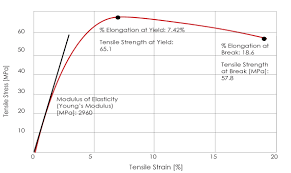## How to Calculate and Solve for Relationship between Modulus of Elasticity on Volume Fraction Porosity, E | CeramicsThe image above represents modulus of elasticity.

To compute for the relationship between modulus of elasticity on volume fraction porosity, two essential parameters are needed and these are Modulus of Elasticity of Non Porous Material (Eo) and Volume Fraction Porosity (P).

The formula for calculating the modulus of elasticity:

E = Eo(1 – 1.9P + 0.9P²)

Where:

E = Modulus of Elasticity
Eo = Modulus of Elasticity of Non Porous Material.
P = Volume Fraction Porosity

Let’s solve an example;
Find the modulus of elasticity when the modulus of elasticity of non porous material is 12 and volume fraction porosity is 22.

This implies that;

Eo = Modulus of Elasticity of Non Porous Material = 12
P = Volume Fraction Porosity = 22

E = Eo(1 – 1.9P + 0.9P²)
E = (12)(1 – 1.9(22) + 0.9(22)²)
E = (12)(1 – (41.8) + 0.9(484))
E = (12)(1 – (41.8) + (435.6))
E = (12)(394.8)
E = 4737.6

Therefore, the modulus of elasticity is 4737.6 Pa.